ARMProgram.com Trend Top Navigation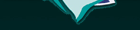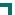Enterococcus faecalis (NORTHCENTRAL Data) 2004 2005 2006 2007 2008 2009 2010 penicillin 96.8%n=666 97.9%n=327 99%n=300 97.7%n=307 99.1%n=320 96.2%n=666 ampicillin 98.4%n=305 98.5%n=327 98.2%n=168 98.5%n=404 99.2%n=389 95.5%n=756 vancomycin 98.3%n=666 99.1%n=327 98.2%n=168 97.8%n=404 99.5%n=389 98.4%n=756 quinupristin/dalfopristin 6.1%n=165 1.2%n=161 1%n=97 1.4%n=69 0%n=90 ciprofloxacin 37%n=165 52.2%n=161 levofloxacin 46.6%n=305 56.6%n=327 64%n=300 62.9%n=404 59.4%n=69 55.6%n=90 nitrofurantoin 100%n=165 100%n=161 98.9%n=666 tetracycline 38.8%n=165 32.3%n=161 28.8%n=264 18.8%n=69 31.1%n=90 gentamicin 24.2%n=165 16.8%n=161 imipenem 100%n=55 100%n=90 rifampim 81.8%n=165 83.9%n=161 Totals 75.6%n=2932 70.9%n=2274 87.5%n=936 75.7%n=1880 88.2%n=1360 91.6%n=3204

This data is accurate as of: 11/22/2016 11:47:41 AM

Drugs with less than 10 isolates are removed from the chart and from the graph. The following drugs had some data removed: quinupristin/dalfopristin, levofloxacin, tetracycline, imipenem.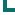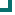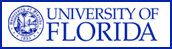ARMProgram.com is a project run by the University of Florida. Copyright 2011. All Rights Reserved. Site by JMarc Technologies, LLC